# Autoencoders for Dimensionality Reduction using TensorFlow in Python

Learn how to benefit from the encoding/decoding process of an autoencoder to extract features and also apply dimensionality reduction using Python and Keras all that by exploring the hidden values of the latent space.
6 min read · Updated aug 2022 · Machine Learning

In this article, we will aim to understand better the capabilities offered by Autoencoders and, more precisely, to explore the latent space. We will use the latter to perform feature extraction and dimensionality reduction. The implementation will be conducted using the Keras Functional API in Tensorflow 2.

The following is the command to install the required libraries for this tutorial:

``\$ pip3 install pandas sklearn tensorflow``

If you have trouble installing TensorFlow, head to this page.

## What is an Autoencoder?

Autoencoders are a type of neural network leveraged for unsupervised learning purposes that try to optimize a set of parameters to compress the input data into the latent space, spot patterns and anomalies, and improve comprehension of the behavior of the data as a whole.

Autoencoders are divided into two parts: an encoder and a decoder; they are used to perform "representation learningwhich is a type of learning that enables a system to find the representations necessary for feature detection or classification from raw data using a class of machine learning techniques. Allowing a model to learn the features and apply them to a specific activity reduces the need for manual feature engineering.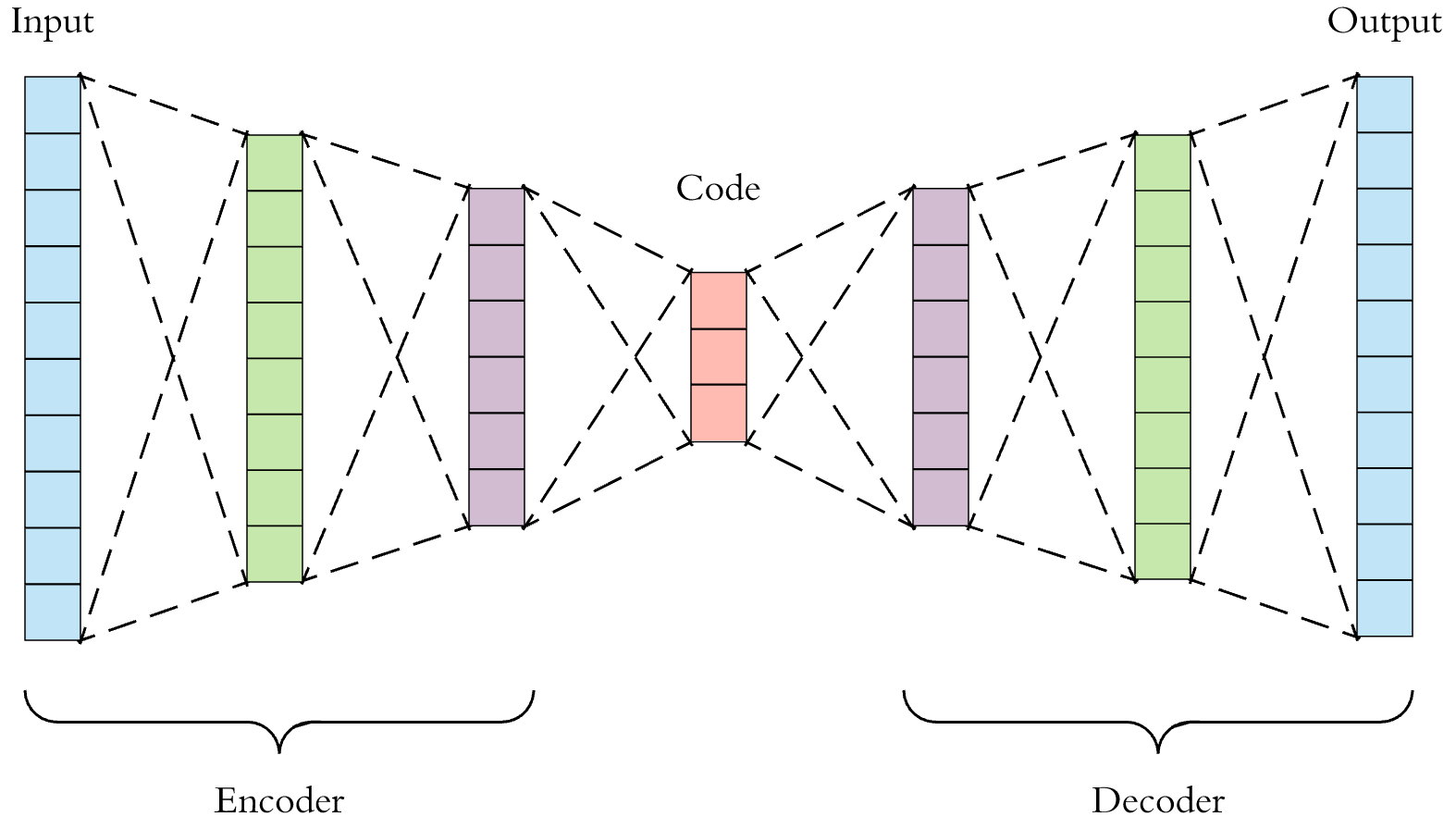Image Source

In representation learning, data is provided to the model, automatically learning the representation. The predictive model's performance is determined by how the features, distance function, and similarity function are represented in the data. Representation learning reduces high-dimensional data to low-dimensional data, which makes it simpler.

Different Use Cases of Autoencoders

In the industry, autoencoders are leveraged to solve different tasks, and some of them will be listed here:

• Denoising Autoencoders: such a model is used when we want to clean the input from some noisy patterns. It is trained by corrupting the input (using Gaussian noise, for instance) and using the original input (before noise introduction) as output.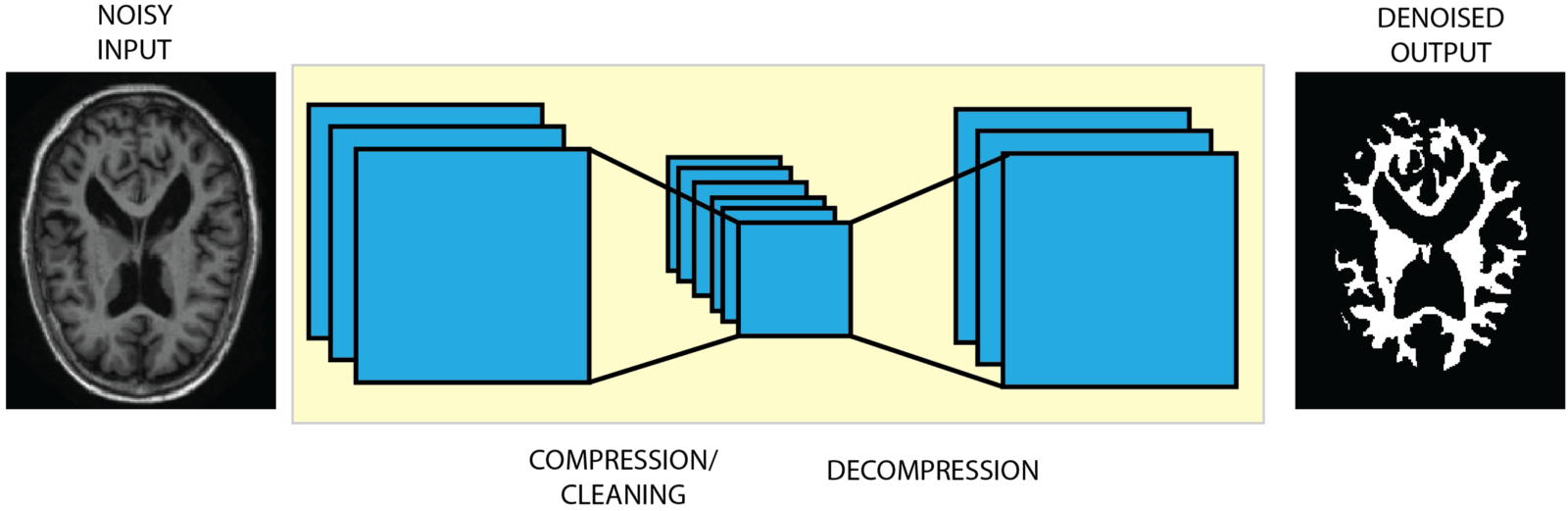Source: Deep AI Denoising AU

• Generation: a flavor of autoencoders called Variational Autoencoder (VAE) learns a probability distribution function to sample and generate new data.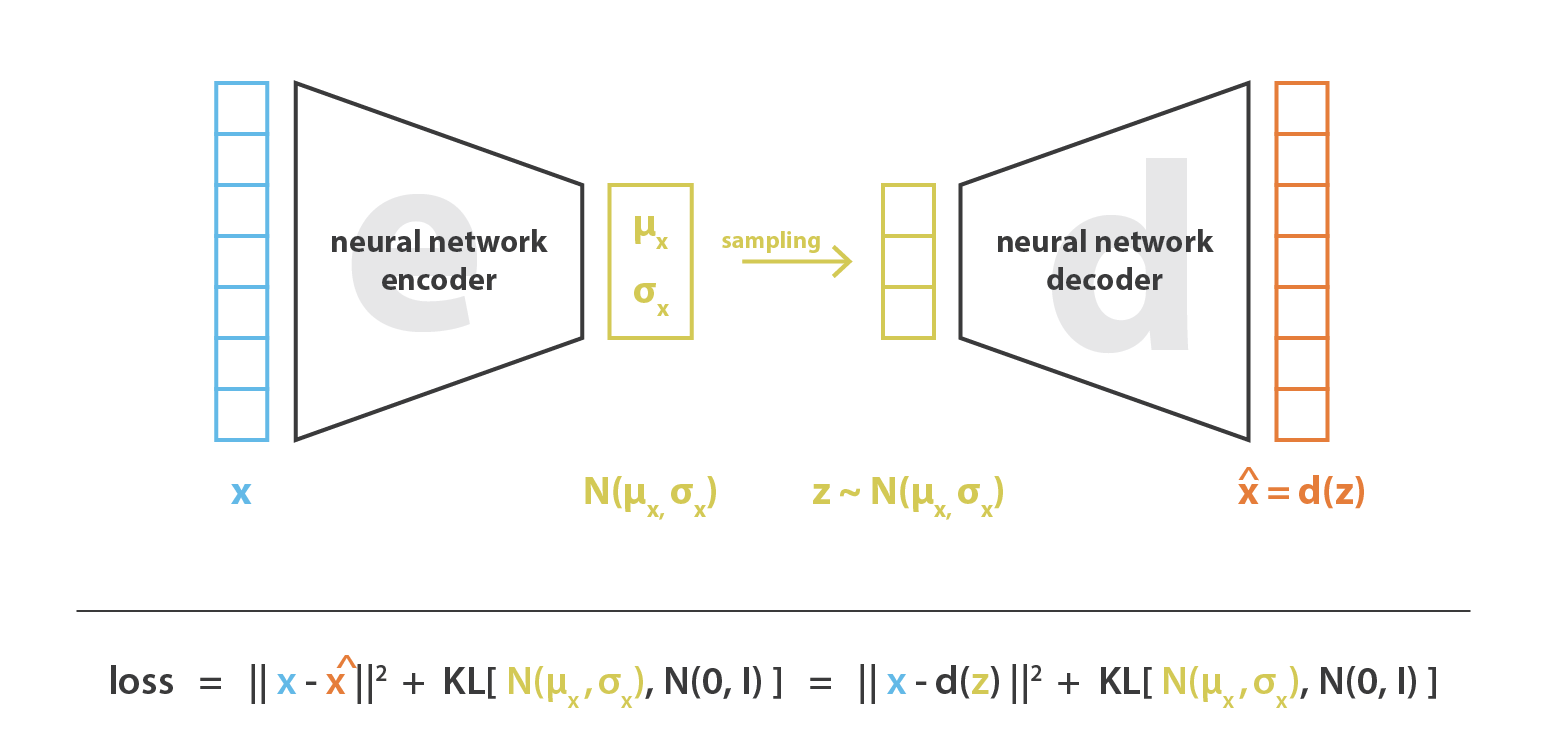Source: Towards Data Science

• Dimensionality Reduction & Feature Selection: This article will further explore this.

What is the Latent Space?

It is the compressed feature space that contains the most relevant portions of the observed data and is located as the intermediate point between the encoder and decoder.

A latent space is formerly described as an abstract, multidimensional space that stores a valuable internal representation of events perceived from the outside. In the latent space, samples that resemble one another externally are placed next to one another.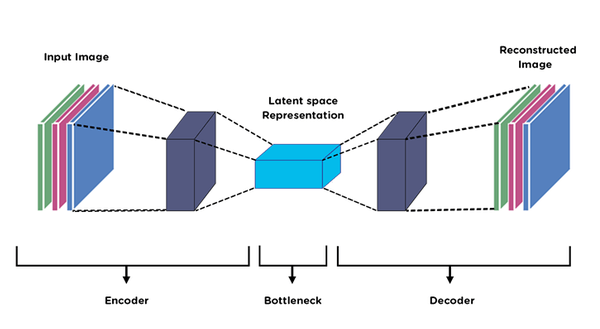Source: Medium

## Used Dataset

Within the scope of our tutorial, we will use the data from Kaggle's IOT Botnets Attack Detection Dataset, and we will more precisely be using the CSV files named `gafgyt_danmini_doorbell_train.csv` and `gafgyt_danmini_doorbell_test.csv`.

## Implementation

We will need the following imports:

``````import pandas as pd
from sklearn.preprocessing import MinMaxScaler
from tensorflow.keras.models import Model
from tensorflow.keras.layers import Input, Dense, LeakyReLU``````
• We will use Pandas to read the data and separate the feature columns from the label column.
• Sklearn will be used to preprocess and normalize the data.
• Keras will be used to build the autoencoder and then keep the encoder part for the feature extraction process.

For the preprocessing, we will apply `MinMaxScaler` normalization as presented here:

``````# Reading Data
# Keeping only features columns for the train set
df_features = df.loc[:, df.columns != "target"]
y_train = df.target
# Keeping only features for the test set
df_features_test = df_test.loc[:, df_test.columns != "target"]
y_test = df_test.target
# Applying the normalization on the train then test set
scaler = MinMaxScaler()
df_features = scaler.fit_transform(df_features)
df_features_test = scaler.transform(df_features_test)``````

## Difference between Sequential and Functional API in Keras

The most straightforward way of creating models in Keras is by using the Sequential API imported from `tensorflow.keras.models` and it allows the user to just stack layers (from `tensorflow.keras.layers`) directly on each other.

Such a method is beneficial for creating fast and easy-to-use deep learning models and if we only want to have a high-level/abstract view of what's happening within the layers.

However, to have more flexibility in the interaction between layers and/or exploiting certain blocks of the model, we should leverage the functional API property of Keras.

The general format of the Functional API in Keras is the following: `output_layer = type_layer(layer_hyperparameters)(input_layer)`

Following this logic, the autoencoder is implemented as follows:

``````# Implementation of the Autoencoder Model
# input from df_features, dense64, leakyrelu, dense32, leakyrelu, dense16, tanh
input = Input(shape=df_features.shape[1:])
enc = Dense(64)(input)
enc = LeakyReLU()(enc)
enc = Dense(32)(enc)
enc = LeakyReLU()(enc)
# latent space with tanh
latent_space = Dense(16, activation="tanh")(enc)

dec = Dense(32)(latent_space)
dec = LeakyReLU()(dec)
dec = Dense(64)(dec)
dec = LeakyReLU()(dec)

dec = Dense(units=df_features.shape, activation="sigmoid")(dec)
# init model
autoencoder = Model(input, dec)
# compile model
# train model
autoencoder.fit(df_features, df_features, epochs=50, batch_size=32, validation_split=0.25)``````

Now, to retrieve the features learned until the latent space, we need to create a model up until the encoder part, which is done as follows:

``encoder = Model(input, latent_space)``

Applying Feature Extraction & Dimensionality Reduction

Let us now try to apply the inference and extract features from the test set.

``test_au_features = encoder.predict(df_features_test)``

And if we want to check the shape of it, we will notice that it has 16 features as the number of units the latent space layer has (`Dense(16)`).

``print(test_au_features.shape)``

Output:

``(15647, 16)``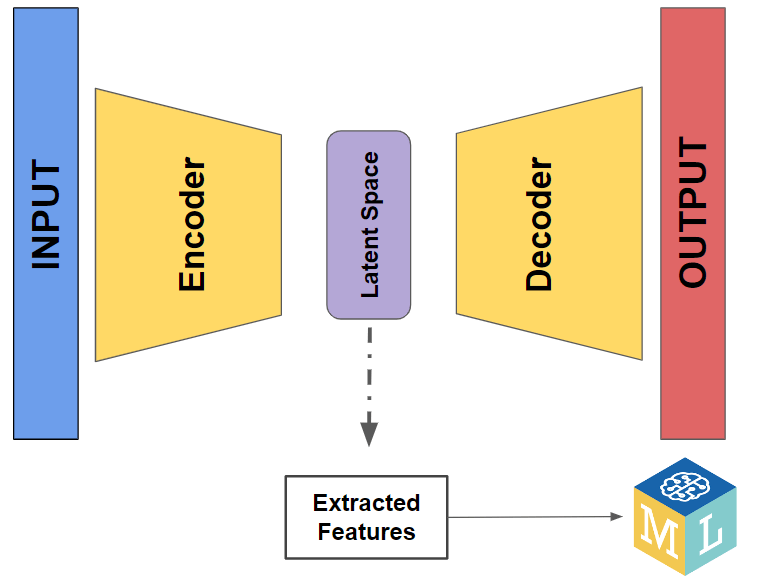Conclusion

• What is an Autoencoder, and its different use cases.
• Difference between Sequential API and Functional API in Keras.
• Trained an autoencoder and then used its trained encoding part to extract features.

Happy learning ♥

Sharing is caring!

### Comment panel